# 1 2 Using a Scientific Approach You are

• Slides: 29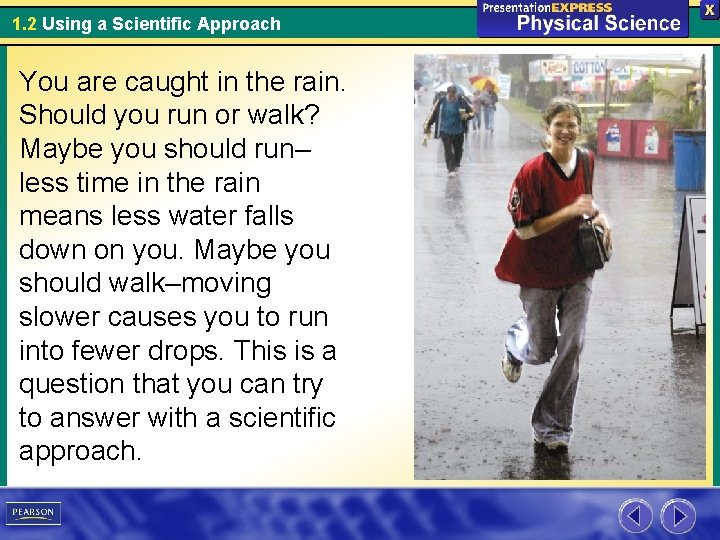1. 2 Using a Scientific Approach You are caught in the rain. Should you run or walk? Maybe you should run– less time in the rain means less water falls down on you. Maybe you should walk–moving slower causes you to run into fewer drops. This is a question that you can try to answer with a scientific approach.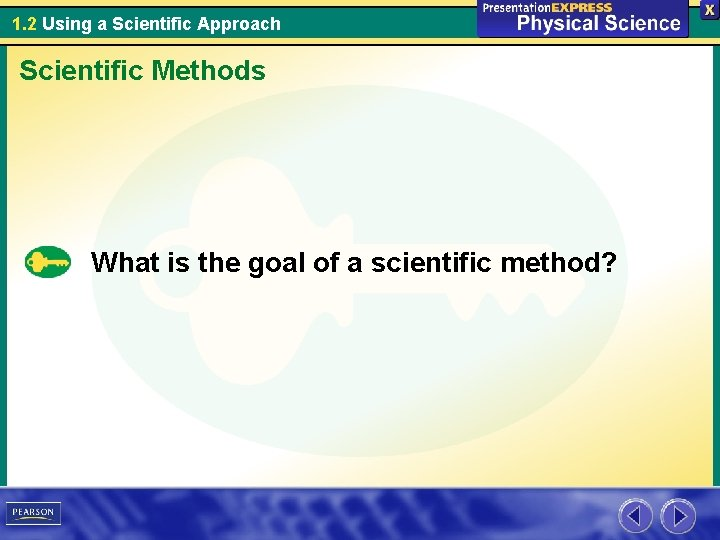1. 2 Using a Scientific Approach Scientific Methods What is the goal of a scientific method?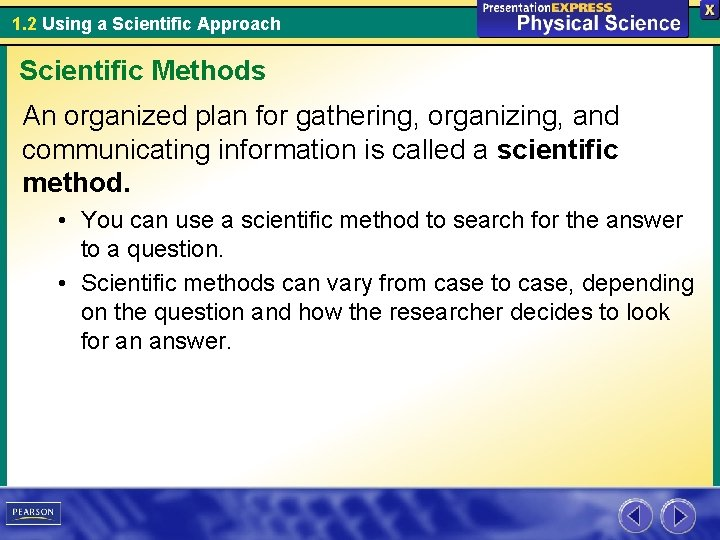1. 2 Using a Scientific Approach Scientific Methods An organized plan for gathering, organizing, and communicating information is called a scientific method. • You can use a scientific method to search for the answer to a question. • Scientific methods can vary from case to case, depending on the question and how the researcher decides to look for an answer.1. 2 Using a Scientific Approach Scientific Methods The goal of any scientific method is to solve a problem or to better understand an observed event.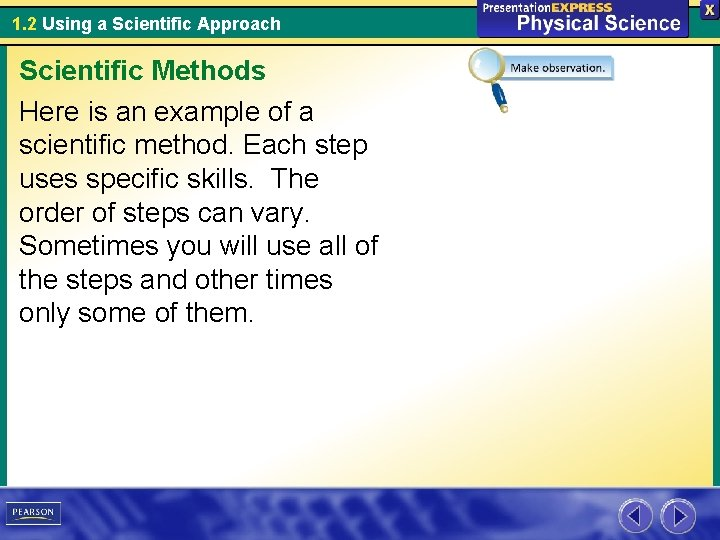1. 2 Using a Scientific Approach Scientific Methods Here is an example of a scientific method. Each step uses specific skills. The order of steps can vary. Sometimes you will use all of the steps and other times only some of them.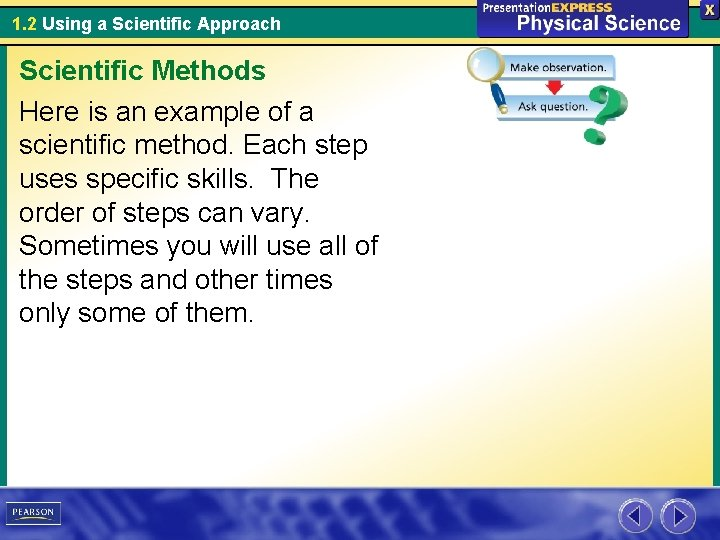1. 2 Using a Scientific Approach Scientific Methods Here is an example of a scientific method. Each step uses specific skills. The order of steps can vary. Sometimes you will use all of the steps and other times only some of them.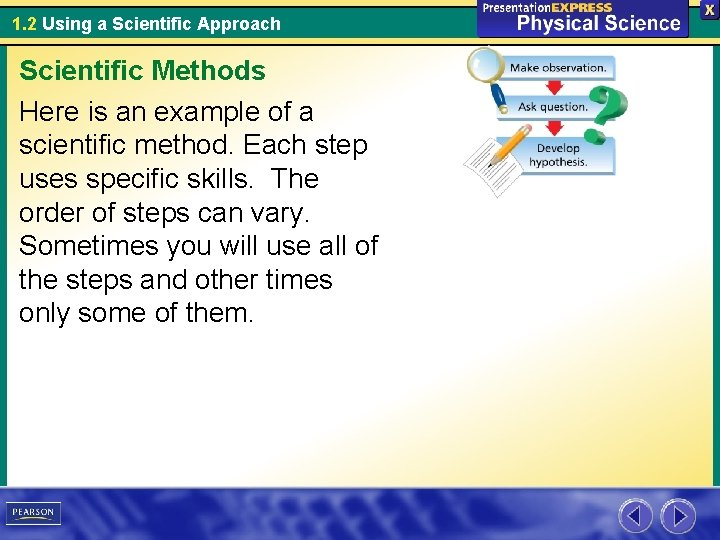1. 2 Using a Scientific Approach Scientific Methods Here is an example of a scientific method. Each step uses specific skills. The order of steps can vary. Sometimes you will use all of the steps and other times only some of them.1. 2 Using a Scientific Approach Scientific Methods Here is an example of a scientific method. Each step uses specific skills. The order of steps can vary. Sometimes you will use all of the steps and other times only some of them.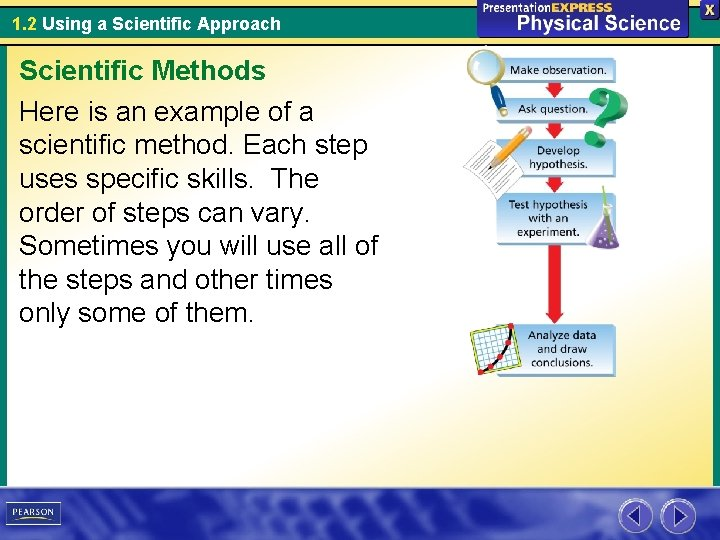1. 2 Using a Scientific Approach Scientific Methods Here is an example of a scientific method. Each step uses specific skills. The order of steps can vary. Sometimes you will use all of the steps and other times only some of them.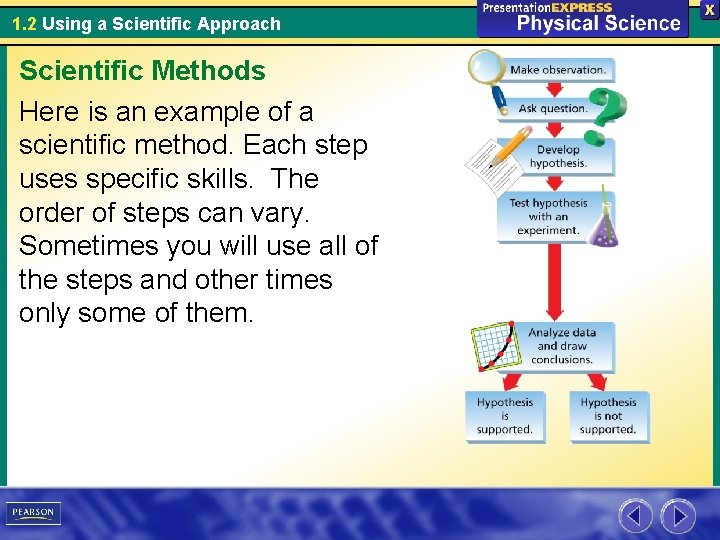1. 2 Using a Scientific Approach Scientific Methods Here is an example of a scientific method. Each step uses specific skills. The order of steps can vary. Sometimes you will use all of the steps and other times only some of them.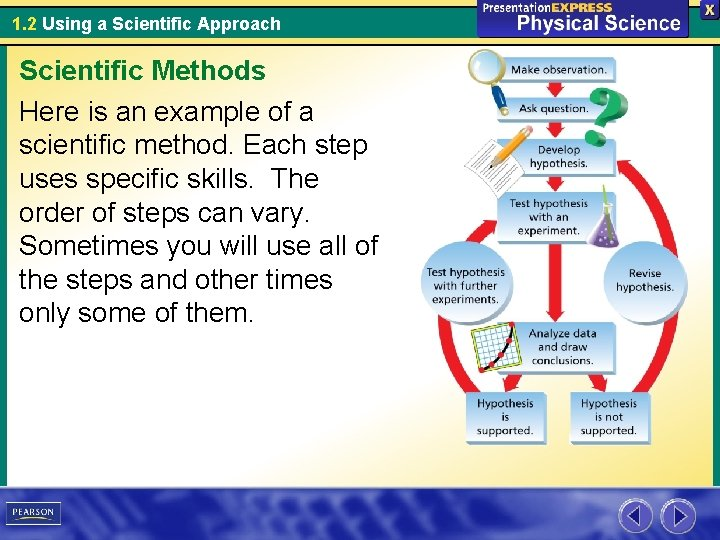1. 2 Using a Scientific Approach Scientific Methods Here is an example of a scientific method. Each step uses specific skills. The order of steps can vary. Sometimes you will use all of the steps and other times only some of them.1. 2 Using a Scientific Approach Scientific Methods Here is an example of a scientific method. Each step uses specific skills. The order of steps can vary. Sometimes you will use all of the steps and other times only some of them.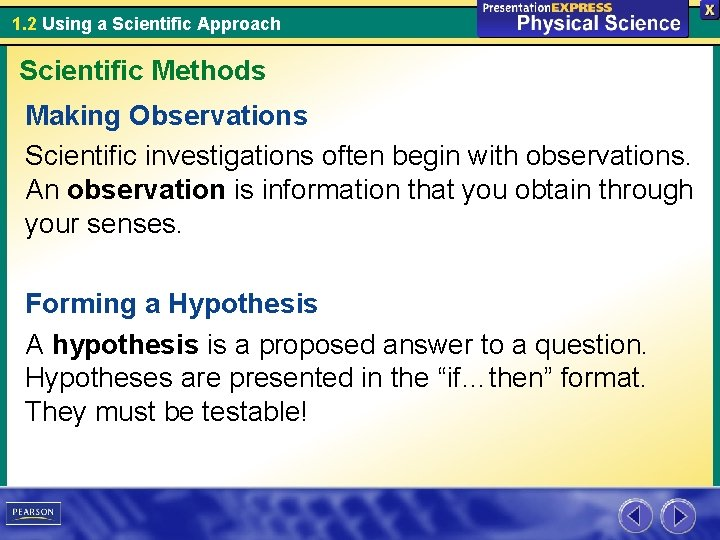1. 2 Using a Scientific Approach Scientific Methods Making Observations Scientific investigations often begin with observations. An observation is information that you obtain through your senses. Forming a Hypothesis A hypothesis is a proposed answer to a question. Hypotheses are presented in the “if…then” format. They must be testable!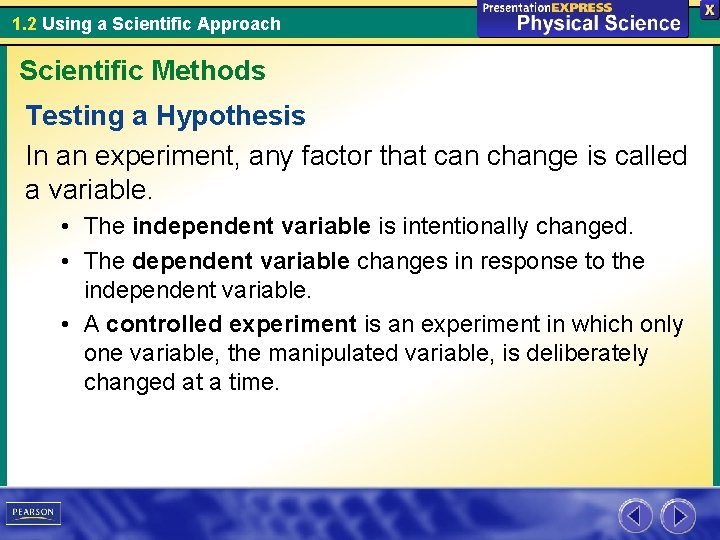1. 2 Using a Scientific Approach Scientific Methods Testing a Hypothesis In an experiment, any factor that can change is called a variable. • The independent variable is intentionally changed. • The dependent variable changes in response to the independent variable. • A controlled experiment is an experiment in which only one variable, the manipulated variable, is deliberately changed at a time.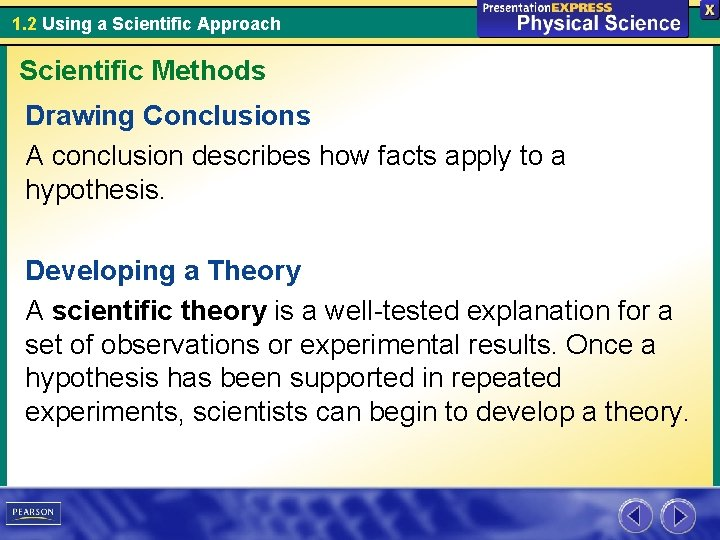1. 2 Using a Scientific Approach Scientific Methods Drawing Conclusions A conclusion describes how facts apply to a hypothesis. Developing a Theory A scientific theory is a well-tested explanation for a set of observations or experimental results. Once a hypothesis has been supported in repeated experiments, scientists can begin to develop a theory.1. 2 Using a Scientific Approach Scientific Methods Question: How does speed affect how wet you get in the rain? Hypothesis: If you run faster in the rain, then you will stay drier. Experiment: Test whether speed affects how wet you get in the rain.1. 2 Using a Scientific Approach Scientific Methods In 1997, two meteorologists conducted a controlled experiment to determine if moving faster keeps you drier in the rain. One scientist walked 100 yards and the other ran the same distance. Variables, such as type of clothes, were controlled.1. 2 Using a Scientific Approach Scientific Methods The clothes of the walking scientist accumulated 217 grams of water; the clothes of the running scientist accumulated 130 grams of water. Draw a Conclusion: The scientists concluded that running in the rain keeps you drier.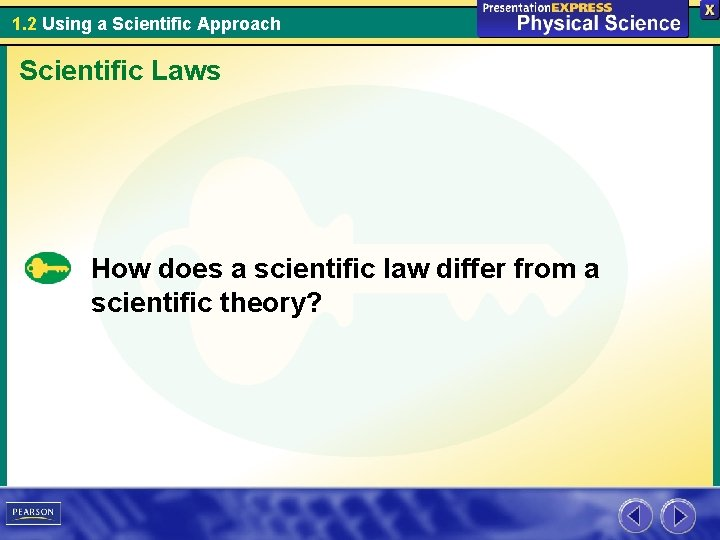1. 2 Using a Scientific Approach Scientific Laws How does a scientific law differ from a scientific theory?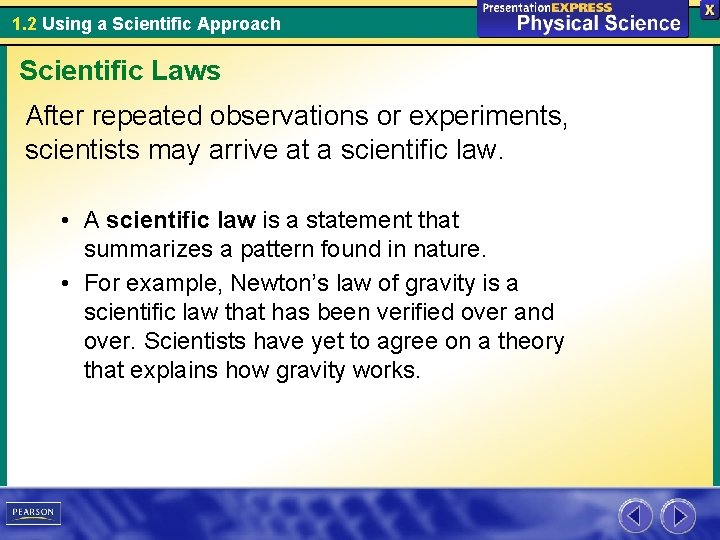1. 2 Using a Scientific Approach Scientific Laws After repeated observations or experiments, scientists may arrive at a scientific law. • A scientific law is a statement that summarizes a pattern found in nature. • For example, Newton’s law of gravity is a scientific law that has been verified over and over. Scientists have yet to agree on a theory that explains how gravity works.1. 2 Using a Scientific Approach Scientific Laws A scientific law describes an observed pattern in nature without attempting to explain it. The explanation of such a pattern is provided by a scientific theory.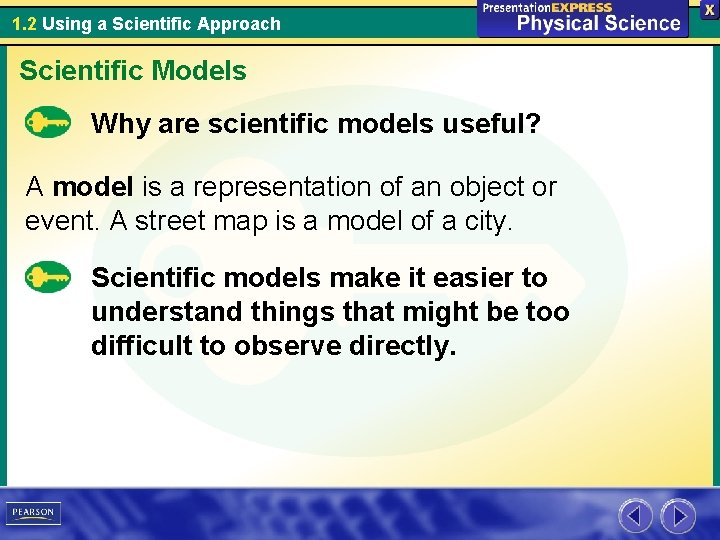1. 2 Using a Scientific Approach Scientific Models Why are scientific models useful? A model is a representation of an object or event. A street map is a model of a city. Scientific models make it easier to understand things that might be too difficult to observe directly.1. 2 Using a Scientific Approach Scientific Models This computer model represents the interior of an airplane. It helps the engineers visualize the layout of the plane.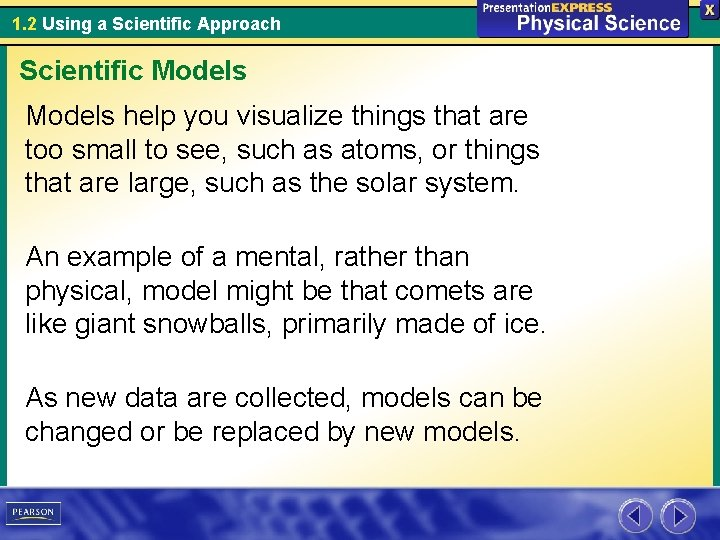1. 2 Using a Scientific Approach Scientific Models help you visualize things that are too small to see, such as atoms, or things that are large, such as the solar system. An example of a mental, rather than physical, model might be that comets are like giant snowballs, primarily made of ice. As new data are collected, models can be changed or be replaced by new models.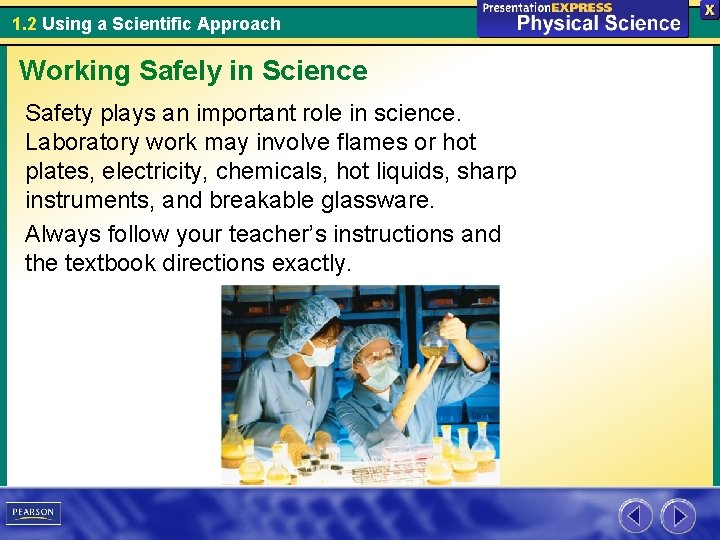1. 2 Using a Scientific Approach Working Safely in Science Safety plays an important role in science. Laboratory work may involve flames or hot plates, electricity, chemicals, hot liquids, sharp instruments, and breakable glassware. Always follow your teacher’s instructions and the textbook directions exactly.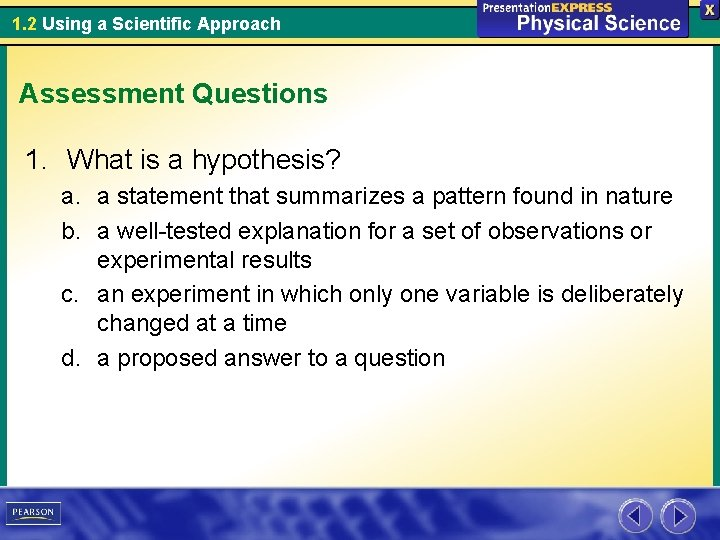1. 2 Using a Scientific Approach Assessment Questions 1. What is a hypothesis? a. a statement that summarizes a pattern found in nature b. a well-tested explanation for a set of observations or experimental results c. an experiment in which only one variable is deliberately changed at a time d. a proposed answer to a question1. 2 Using a Scientific Approach Assessment Questions 2. How does a scientific law differ from a theory? a. A scientific law describes observations while a theory provides an explanation. b. A scientific law cannot be changed by additional observations, but a theory can be changed. c. A theory is a possible explanation, but a scientific law is a proven explanation. d. There is no difference because scientific law and theory are two terms that mean the same thing.1. 2 Using a Scientific Approach Assessment Questions 3. What is the main purpose of a scientific model? a. b. c. d. to show a hypothesis was developed to prove a theory to make complex concepts easier to understand to show a large object, such as the Earth, in a smaller form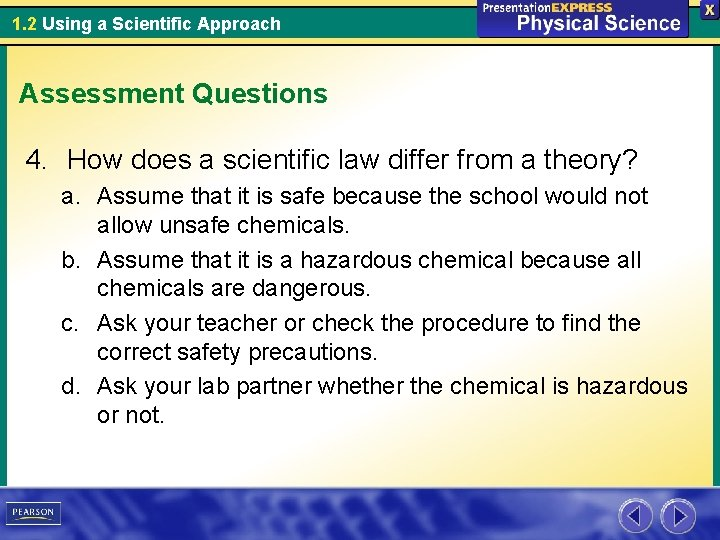1. 2 Using a Scientific Approach Assessment Questions 4. How does a scientific law differ from a theory? a. Assume that it is safe because the school would not allow unsafe chemicals. b. Assume that it is a hazardous chemical because all chemicals are dangerous. c. Ask your teacher or check the procedure to find the correct safety precautions. d. Ask your lab partner whether the chemical is hazardous or not.